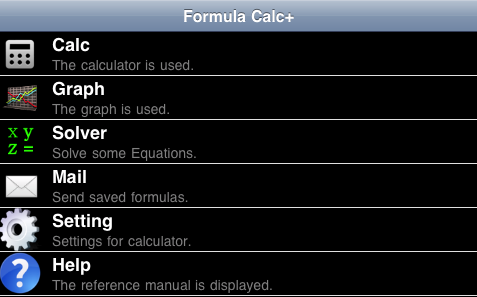# Reference Manual

## Abstract

• This calculator can be calculated by inputting the formula unlike a usual calculator.
• The variable can be used in the formula. You can use alphabet(a-z) and greek alphabet(α-ω) as the variable.
• You can edit formula with moving caret.
• You can create the graph by using the created formula.
• You can send the created formula with mail.
• You can solve some equations.
Please refer to the following section for details.